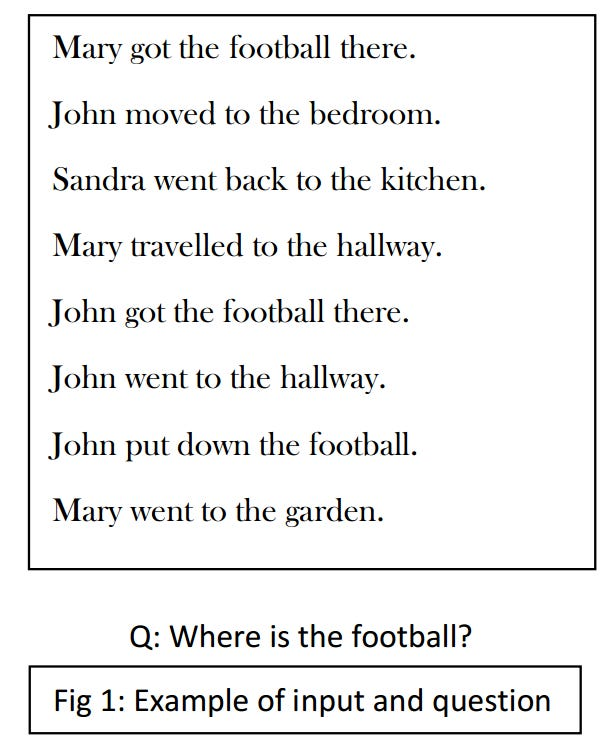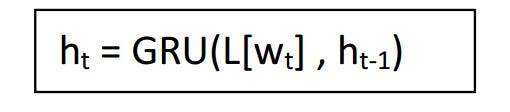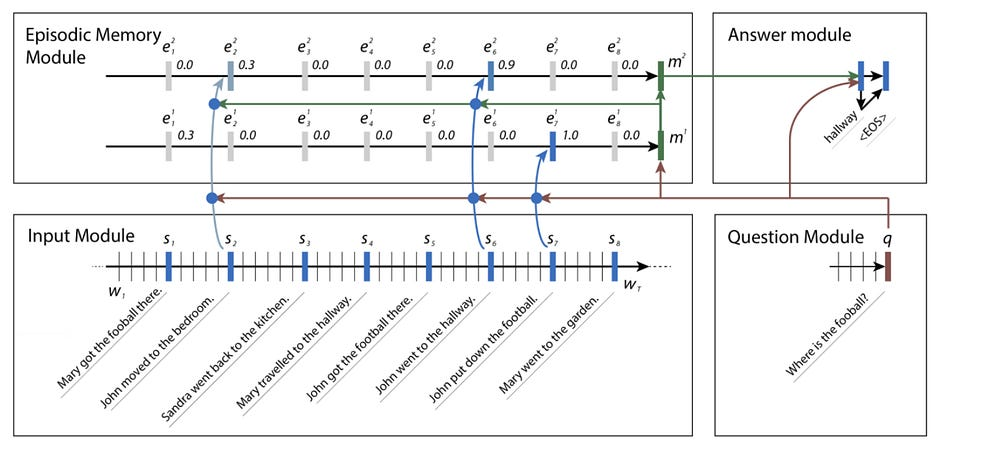# Dynamic Memory Networks

Source: Deep Learning on Medium

Human beings communicate in a complex and a detailed way unlike most other living beings. The ability of raising questions and answering them enables us to acquire knowledge and process learning. Now replicating such behaviour using artificial intelligence is not going to be an easy feat. It involves both the skill to understand text as well as reason the facts properly. One such effort towards understanding and reasoning is through Dynamic Memory Networks — a general neural-network based architecture that is trained on input-question-answer triplets. Most of the tasks could be reduced to a question-answering problem. For example, we can think of an image
classification problem as asking a question like “What class does this image belong to?” or a machine translation problem as asking, “What is the translation of this in French?”
Let’s get an idea of how these networks look and what can they achieve. Dynamic Memory
Networks(DMN) are comprised of four modules.
1. Input module
2. Question module
3. Episodic memory module
4. Answer module
We will walk through an example to see what each module contributes. Let’s say we have the text and question asFigure 1: Example

I’m sure it is not hard for our human brains to decode that since John went to the hallway and John put down the football, the football must have been in the hallway. If you notice, what our brain is trying to do appears to be transitive learning by connecting John <-> hallway and John <-> football to
obtain a logic as hallway <-> football.

Let’s see why a machine needs four modules to achieve this task while our brain did so effortlessly.

Input module: We need to able to convert the input given above in a way that our model understands. In natural language processing problems, we use word vector representations. Here, the sequence of words is converted to their word vector representations and given as inputs to a recurrent neural network. For the experiments given in the paper, a gated recurrent network (GRU)
is used. This choice is made after exploring LSTM and tanh RNN because of lesser computational cost and lesser suffering from vanishing gradient problem respectively.

The sentences are first concatenated into a long list of word tokens inserting an EOS token.where L is an embedding matrix and wt is the word index of tth word of the input sequence.

where L is an embedding matrix and wₜ is the word index of tᵗʰ word of the input sequence.

The hidden states at each end-of-sentence tokens are the final representations of this module. The output of the input module is a sequence of T𝒸 fact representations c where cₜ denotes the tᵗʰ element in the output sequence. In our case, T𝒸 = 8 since we have 8 sentences in our input text.

Question module: Since the question is also a natural language input, it is encoded similar to what is done in the input module.

The output of this module is a final representation q(T) where T=T(Q)

Episodic memory module: This module is the heart of this paper. It consists of an attention mechanism and an RNN to update its memory in multiple passes. During each iteration of the RNN, the attention mechanism attends over the fact representations by taking into consideration the question representation q and the previous memory mᵢ₋₁ to produce an episode eᵢ which is then used to update memory. The memory m₀ is initialized to question module output q.

For certain tasks, it is beneficial to take multiple passes over the input to allow transitive inference. In our example, in the first pass, in the context of the question asked Where is the football? , the model attends sentence 7 — John put down the football because of the word football but in order to answer where, it needs additional information and hence requires another pass. In the second iteration, attention is performed with the respect to the word where in the question along with the previous memory that tells us that John and football are related.

After multiple such passes, the final memory m(Tᴹ) is passed to the answer module.

Let’s have a closer look at how attention mechanism works. For each pass i, this takes as input cₜ, previous memory mᵢ₋₁ and question q to compute a gate. The gating function G is computed as

The score function z captures a sense of similarity between the input, question and previous memory. In the memory update mechanism, to compute the episode eᵢ for pass i, a GRU is used over the sequence of inputs weighted by gates gₜ(i)

Answer module: The output of the answer module depends on the type of task we would like to perform. Since in the example taken, we would like to generate a textual answer, an answer module is triggered at the end of the episodic memory (unlike other cases which can trigger it at each time
step of episodic memory)

Another GRU is employed whose hidden state at is initialised to m(Tᴹ) and yₜ is computed in the following way.

The last generated word and the question vector are concatenated and sent as input at each time step. The output so obtained is trained with a cross-entropy loss.Figure 2: DMN Architecture

PS: The results can be referred from the original paper.

Ankit Kumar, Peter Ondruska, Mohit Iyyer, James Bradbury, Ishaan Gulrajani, Victor Zhong, Romain Paulus, Richard Socher. 2016 “Ask Me Anything: Dynamic Memory Networks for Natural Language Processing”.CoRR.